U.S. Department of Transportation
1200 New Jersey Avenue, SE
Washington, DC 20590
202-366-4000

Federal Highway Administration Research and Technology
Coordinating, Developing, and Delivering Highway Transportation InnovationsThis report is an archived publication and may contain dated technical, contact, and link information
 Federal Highway Administration > Publications > Research Publications > LTPP Publications > 03041 > Appb.Cfm Evaluation and Analysis of LTPP Pavement Layer Thickness Data
 Publication Number: FHWA-RD-03-041

# Evaluation and Analysis of LTPP Pavement Layer Thickness Data

### Statistical Formulations Used in the Skewness and Kurtosis Test

The following formulations for the combined skewness and kurtosis test were developed based on the reference .

For the skewness, we have: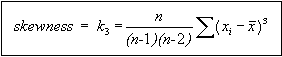Figure 76: Equation. Skewness definition.

For kurtosis, we have: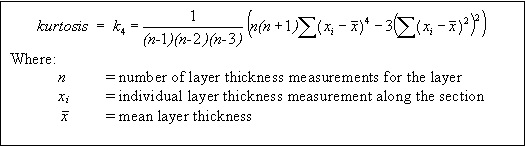Figure 77: Equation. Kurtosis definition.

To evaluate the skewness and kurtosis tests results, the non-dimensional skewness and kurtosis coefficients are computed, as following:Figure 78: Equation. Non-dimensional skewness coefficient definition.Figure 79: Equation. Non-dimensional kurtosis coefficient definition.

Based on the g1 and g2 values, the statistics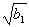and b2 are found next: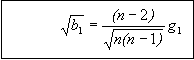Figure 80: Equation. Definition ofstatistic.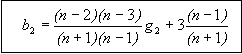Figure 81: Equation. Definition of b2 statistic.

To find z1 value, the following parameters are computed usingand b2 statistics: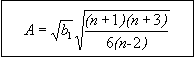Figure 82: Equation. Definition of intermediate parameter A.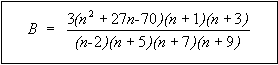Figure 83: Equation. Definition of intermediate parameter B.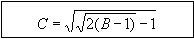Figure 84: Equation. Definition of intermediate parameter C.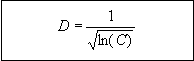Figure 85: Equation. Definition of intermediate parameter D.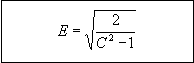Figure 86: Equation. Definition of intermediate parameter E.

The corresponding z1 value used as a skewness test statistic is the following: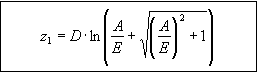Figure 87: Equation. Definition of skewness test statistic z1.

To find z2 value, the following intermediate parameters are computed next: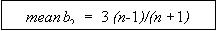Figure 88: Equation. Definition of the mean of intermediate parameter meanb2.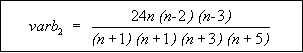Figure 89: Equation. Definition of the variance of intermediate parameter varb2.Figure 90: Equation. Definition of intermediate parameter F.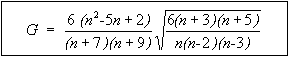Figure 91: Equation. Definition of intermediate parameter G.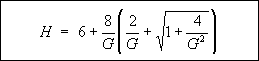Figure 92: Equation. Definition of intermediate parameter H.

The corresponding z2 value used as a kurtosis test statistic is the following: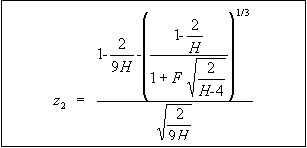Figure 93: Equation. Definition of kurtosis test statistic z2.

The z1 and z2 statistics are used to obtain the p-values (the probability that values of the standard normal distribution are more extreme than the computed z1 and z2 statistics).